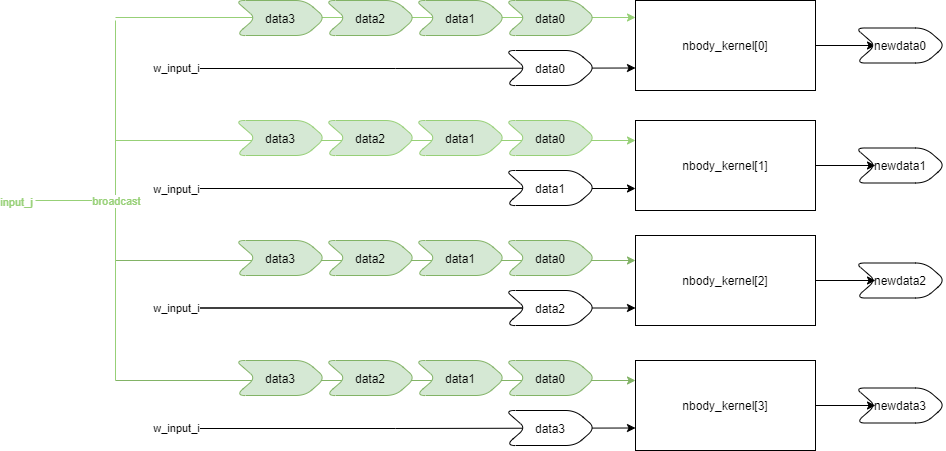# Workload Distribution and input_j - 2022.2 English

## Vitis Tutorials: AI Engine Development

Document ID
XD100
Release Date
2022-12-01
Version
2022.2 English

To calculate the N-Body gravity equations for 128 particles, each `nbody()` kernel calculates the N-Body gravity equations for 32 particles. However, in order to calculate acceleration and the new velocities, an `nbody()` kernel needs to know the data in the other kernels. For example, if particle 0 is mapped to `nbody_kernel` and particle 32 is mapped to `nbody_kernel`, then `nbody_kernel` needs to know the data in `nbody_kernel` to accurately calculate the summation equation for acceleration and then calculate the new velocity of particle 0.

This is where the `input_j` stream plays a vital role in data sharing. Even though the `input_j` data stream has a window size for 32 particles worth of data, the `LOOP_COUNT_J` value can be set to allow the `nbody()` kernels to take in any number of 32 particles worth of data at a time. For a single instance of the `nbody_subsystem` graph, the `LOOP_COUNT_J` should be set to 4 to stream in data for all four kernels. For the final AI Engine graph, which contains 100 instances of the `nbody_subsystem` graph, the `LOOP_COUNT_J` value is set to 400 to stream in data for all 400 kernels to each `nbody()` kernel.For example, to calculate the new velocity of particle 0 mapped in `nbody_kernel`, the `nbody_kernel` can retrieve the data value of particle 32 from the `input_j` stream. This way, all `nbody()` kernels will have the data values for all other particles mapped in the other `nbody()` kernels through data streaming from `input_j`.